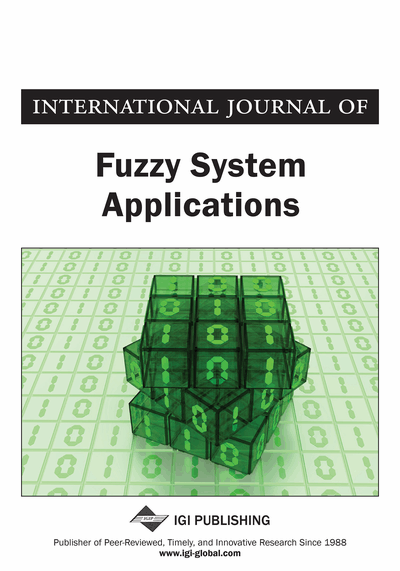# Cost Efficiency Measures with Trapezoidal Fuzzy Numbers in Data Envelopment Analysis Based on Ranking Functions: Application in Insurance Organization and Hospital

DOI: 10.4018/ijfsa.2012070104
Available
\$29.50
No Current Special Offers

## Abstract

Cost efficiency (CE) evaluates the ability to produce current outputs at minimal cost, given its input prices. In ordinary CE model, the input prices are assumed to be definite. In recent years, various attempts have been made to measuring CE when the input prices are as trapezoidal fuzzy numbers. The main contribution of this paper is to provide a new approach for generalizing the CE of decision making units in data envelopment analysis when the input prices are trapezoidal fuzzy numbers, where concepts of fuzzy linear programming problems and CE, are directly used. Here, the author used the linear ranking functions to compare fuzzy numbers. The proposed method is illustrated with two application examples and proves to be persuasive and acceptable in real world systems.
Article Preview
Top

## Introduction

There are three main topics that are relevant to this paper: Fuzzy data envelopment analysis (DEA), Application of DEA to hospital and insurance organization efficiency assessments and fuzzy linear programming problems.

DEA is a non-parametric technique for evaluating decision making units (DMUs) based on the production possibility set. Also, traditional DEA is used for measuring the efficiency of a set of DMUs where the input and output data of the DMUs are known exactly (Charnes et al., 1978). Entani et al. (2002), Despotis and Smirlis (2002), Zhu (2003a, 2003b), Wang et al. (2005), and Kao (2006) developed the theory of efficiency measurement where the data are imprecise. Saati et al. (2002) presented a fuzzy version of CCR model with a symmetrical triangular fuzzy number and suggested a procedure for its solution. The basic idea was to transform the fuzzy CCR model into a crisp linear programming problem by applying an alternative-cut approach. They also proposed a ranking method for fuzzy DMUs using presented fuzzy DEA approach. Kao and Liu (2000) developed a method to find the membership functions of the fuzzy efficiency scores when some observations are fuzzy numbers. Entani et al. (2002) proposed interval efficiency obtained from the pessimistic and the optimistic viewpoints. Since Zadeh (1965, 1978) initiated the possibility measure, many researchers have introduced it into DEA (Kao, 2000; Guo, 2001). Although possibility measure has been widely used, it has no self-duality property. However, a self-dual measure is absolutely needed in both theory and practice. In order to define a self-dual measure, Liu and Liu (2002) presented the concept of credibility measure. An axiomatic foundation of credibility theory was given by Liu (2004). Wen and Li (2009) employed the credibility measure to the fuzzy DEA models. Moreover, Bagherzadeh Valami (2009) used the preliminary concepts of fuzzy theory and cost efficiency (CE) directly to provide a fuzzy set as the minimum cost for producing the outputs of the target DMU. Finally, he introduced a method for comparing the production cost of the target DMU with the minimum cost fuzzy set. Mostafaee and Saljooghi (2010) proposed a pair of two-level mathematical programming problems to obtain the upper and lower bounds of cost efficiency when some of the input and output data appear in the form of ranges.

## Complete Article List

Search this Journal:
Reset
Volume 12: 1 Issue (2023)
Volume 11: 4 Issues (2022)
Volume 10: 4 Issues (2021)
Volume 9: 4 Issues (2020)
Volume 8: 4 Issues (2019)
Volume 7: 4 Issues (2018)
Volume 6: 4 Issues (2017)
Volume 5: 4 Issues (2016)
Volume 4: 4 Issues (2015)
Volume 3: 4 Issues (2013)
Volume 2: 4 Issues (2012)
Volume 1: 4 Issues (2011)
View Complete Journal Contents Listing